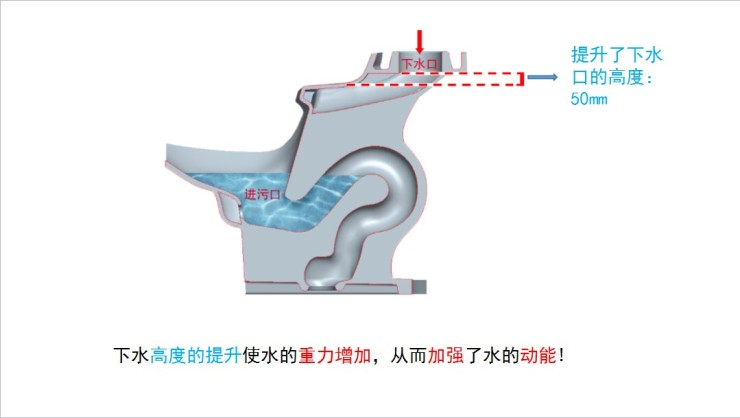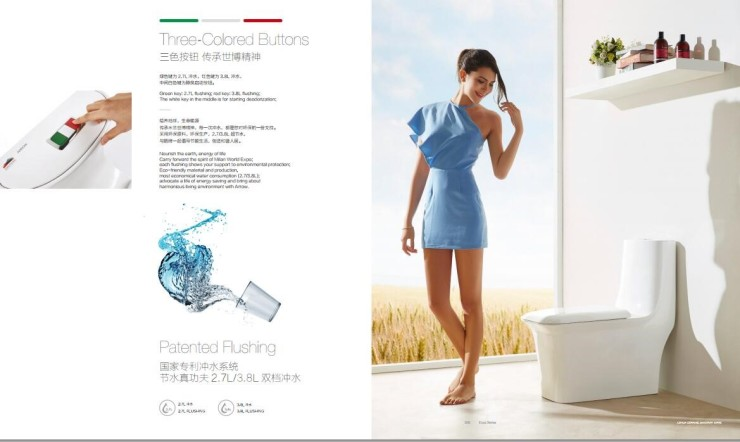|

# 世界水日谈厕所节水?是时候谈下中国的“卫浴革命”了

一年一度的“世界水日”来临，而今年大家关注的点却在“厕所”上？近日，在百度指数上以“世界水日”作为关键词进行搜索，发现受众对世界水日的“需求图谱”上，“厕所”的搜索需求上升趋势明显。

厕所与呼吁“节水”的世界水日相挂靠，或许足以说明，厕所节水及与之相关的卫浴节水正为成全民焦点。

“世界水日”：不得不提中国的厕所节水

“世界水日”的到来，唤起了人们对水资源消耗的关注，而厕所节水与之息息相关，我们每天很习以为常的一个冲马桶动作，其实会对水资源产生很大的浪费。据不完全统计计算，全国冲水马桶每年冲走90亿立方米的水量，相当于九个西湖、两个太湖的水量。

可以说，厕所节水的关键就在于如何实现马桶的节水，这也是中国卫浴企业一直在致力于解决的问题。

民族卫浴行业的龙头企业箭牌卫浴方面便表示，“世界水日”全民关注厕所节水，这背后不仅展现了人们对日常节水问题的重视，更折射了国内卫浴行业节水理念的变革。

以箭牌卫浴马桶的节水技术更新换代为例，据其技术人员介绍，普通马桶在细节设计上不够严谨，只有加大耗水量才能达到冲刷干净的目的，而这无疑会造成水资源的浪费。箭牌卫浴马桶设计图

而箭牌卫浴的节水马桶采用了其自主研发的超节水冲水系统，在节水设计上，一方面是将马桶的下水口的高度提升了50mm，从而使水的重力增加，加大了水冲刷马桶的动能；另一方面，进污口与管道连接处采用“喇叭”形设计，连接处进行了圆弧形过渡处理，可以减少水流流动的阻力，帮助加速水流流动，增加冲水力度，让便便一滑到底。

据了解，一般的节水马桶只能刚好达到国家节水标准，而箭牌卫浴采用“冲吸双擎”劲漩冲洗系统的世博系列马桶产品，其节水标准远优于国家节水标准，能够实现2.7升超级节水。

厕所节水引发卫浴变革？

业界专家分析指出，随着我国对环境友好型社会的提倡、人们环保节水理念的加强，马桶节水技术的更新换代是大势所趋，这背后也折射了整个卫浴行业环保节水的大变革。

当前，市面上除了节水马桶，提倡节水的环保型卫浴产品比比皆是，节水节能、低碳环保也成为人们选购卫浴产品的主要诉求·····

新形势下，卫浴领域其实早已掀起产品“节水环保技能”的大比拼，政府以及行业组织针对卫浴产品的节水评测机制也日趋完善，于是，越来越多的卫浴企业选择踏上环保整改的道路，践行环保节水理念，分担水资源压力。

以业界比较权威的“金马桶”测评及调研活动为例，据了解，这个由中国建筑装饰协会主办，国家节水器具产品质量监督检验中心加以技术支持的行业盛事，每年都会吸引诸多卫浴品牌的加入，卫浴产品技术的比拼，在很大程度上引导着卫浴行业的节水环保技术提升与标准化。箭牌卫浴世博系列马桶产品

2016年，打败诸多竞争对手，摘得“金马桶”桂冠的是箭牌卫浴AB1169坐便器。评测结果显示，其在节水指标、环保指标、卫生（洁净）指标等方面均超过了其他同类产品，平均用水量只有2.6L，远低于国家的5L标准。

除了节水技术的创新，业界专家表示，这次摘得“金马桶”更源于箭牌卫浴自身对环保节水理念的一以贯之。

换句话说，环保节水的理念只有深入卫浴企业发展各阶段、生产制造各环节，有了这样的底气之后，才真正能够实现节水技能的飞跃，在激烈的市场竞争中杀出重围。

据了解，成立于1994年的箭牌卫浴，在创立之初就坚持执行国际质量、环境管理体系标准，近年来，面对日益激烈的市场竞争以及人们对节水卫浴的新需求，箭牌卫浴选择加大产品环保节水技术的创新，并提出了“人文卫浴”的概念，将人性化设计应用到环保节水产品的改进与完善，创造适合中国人的环保型卫浴产品。

回到“世界水日”，每年的3月22日，全世界都会把目光投向水，投向水与人类的生存。而今年，透过“世界水日”人们对厕所节水问题的关注，我们也不难发现，人们的环保节水意识正落实到每时每刻、每天的生活实践里。

再往深处挖，节水意识的向下落实离不开箭牌卫浴这些卫浴企业的努力，只有在生产制造的源头上贯彻环保节约理念，创新卫浴节水环保技术，我们的节水才真正有了“技术依托”。

而全国上下，职责共担，“世界水日”的价值才得以呈现，这些或许值得我们进一步的反思。

`声明：本文由入驻焦点开放平台的作者撰写，除焦点官方账号外，观点仅代表作者本人，不代表焦点立场错误信息举报电话： 400-099-0099，邮箱：jubao@vip.sohu.com，或点此进行意见反馈，或点此进行举报投诉。`A B C D E F G H J K L M N P Q R S T W X Y Z
A - B - C - D - E
• A
• 鞍山
• 安庆
• 安阳
• 安顺
• 安康
• 澳门
• B
• 北京
• 保定
• 包头
• 巴彦淖尔
• 本溪
• 蚌埠
• 亳州
• 滨州
• 北海
• 百色
• 巴中
• 毕节
• 保山
• 宝鸡
• 白银
• 巴州
• C
• 承德
• 沧州
• 长治
• 赤峰
• 朝阳
• 长春
• 常州
• 滁州
• 池州
• 长沙
• 常德
• 郴州
• 潮州
• 崇左
• 重庆
• 成都
• 楚雄
• 昌都
• 慈溪
• 常熟
• D
• 大同
• 大连
• 丹东
• 大庆
• 东营
• 德州
• 东莞
• 德阳
• 达州
• 大理
• 德宏
• 定西
• 儋州
• 东平
• E
• 鄂尔多斯
• 鄂州
• 恩施
F - G - H - I - J
• F
• 抚顺
• 阜新
• 阜阳
• 福州
• 抚州
• 佛山
• 防城港
• G
• 赣州
• 广州
• 桂林
• 贵港
• 广元
• 广安
• 贵阳
• 固原
• H
• 邯郸
• 衡水
• 呼和浩特
• 呼伦贝尔
• 葫芦岛
• 哈尔滨
• 黑河
• 淮安
• 杭州
• 湖州
• 合肥
• 淮南
• 淮北
• 黄山
• 菏泽
• 鹤壁
• 黄石
• 黄冈
• 衡阳
• 怀化
• 惠州
• 河源
• 贺州
• 河池
• 海口
• 红河
• 汉中
• 海东
• 怀来
• I
• J
• 晋中
• 锦州
• 吉林
• 鸡西
• 佳木斯
• 嘉兴
• 金华
• 景德镇
• 九江
• 吉安
• 济南
• 济宁
• 焦作
• 荆门
• 荆州
• 江门
• 揭阳
• 金昌
• 酒泉
• 嘉峪关
K - L - M - N - P
• K
• 开封
• 昆明
• 昆山
• L
• 廊坊
• 临汾
• 辽阳
• 连云港
• 丽水
• 六安
• 龙岩
• 莱芜
• 临沂
• 聊城
• 洛阳
• 漯河
• 娄底
• 柳州
• 来宾
• 泸州
• 乐山
• 六盘水
• 丽江
• 临沧
• 拉萨
• 林芝
• 兰州
• 陇南
• M
• 牡丹江
• 马鞍山
• 茂名
• 梅州
• 绵阳
• 眉山
• N
• 南京
• 南通
• 宁波
• 南平
• 宁德
• 南昌
• 南阳
• 南宁
• 内江
• 南充
• P
• 盘锦
• 莆田
• 平顶山
• 濮阳
• 攀枝花
• 普洱
• 平凉
Q - R - S - T - W
• Q
• 秦皇岛
• 齐齐哈尔
• 衢州
• 泉州
• 青岛
• 清远
• 钦州
• 黔南
• 曲靖
• 庆阳
• R
• 日照
• 日喀则
• S
• 石家庄
• 沈阳
• 双鸭山
• 绥化
• 上海
• 苏州
• 宿迁
• 绍兴
• 宿州
• 三明
• 上饶
• 三门峡
• 商丘
• 十堰
• 随州
• 邵阳
• 韶关
• 深圳
• 汕头
• 汕尾
• 三亚
• 三沙
• 遂宁
• 山南
• 商洛
• 石嘴山
• T
• 天津
• 唐山
• 太原
• 通辽
• 铁岭
• 泰州
• 台州
• 铜陵
• 泰安
• 铜仁
• 铜川
• 天水
• 天门
• W
• 乌海
• 乌兰察布
• 无锡
• 温州
• 芜湖
• 潍坊
• 威海
• 武汉
• 梧州
• 渭南
• 武威
• 吴忠
• 乌鲁木齐
X - Y - Z
• X
• 邢台
• 徐州
• 宣城
• 厦门
• 新乡
• 许昌
• 信阳
• 襄阳
• 孝感
• 咸宁
• 湘潭
• 湘西
• 西双版纳
• 西安
• 咸阳
• 西宁
• 仙桃
• 西昌
• Y
• 运城
• 营口
• 盐城
• 扬州
• 鹰潭
• 宜春
• 烟台
• 宜昌
• 岳阳
• 益阳
• 永州
• 阳江
• 云浮
• 玉林
• 宜宾
• 雅安
• 玉溪
• 延安
• 榆林
• 银川
• Z
• 张家口
• 镇江
• 舟山
• 漳州
• 淄博
• 枣庄
• 郑州
• 周口
• 驻马店
• 株洲
• 张家界
• 珠海
• 湛江
• 肇庆
• 中山
• 自贡
• 资阳
• 遵义
• 昭通
• 张掖
• 中卫

1室1厅1厨1卫1阳台

1
2
3
4
5

0
1
2

1

1

0
1
2
3报名成功，资料已提交审核A B C D E F G H J K L M N P Q R S T W X Y Z
A - B - C - D - E
• A
• 鞍山
• 安庆
• 安阳
• 安顺
• 安康
• 澳门
• B
• 北京
• 保定
• 包头
• 巴彦淖尔
• 本溪
• 蚌埠
• 亳州
• 滨州
• 北海
• 百色
• 巴中
• 毕节
• 保山
• 宝鸡
• 白银
• 巴州
• C
• 承德
• 沧州
• 长治
• 赤峰
• 朝阳
• 长春
• 常州
• 滁州
• 池州
• 长沙
• 常德
• 郴州
• 潮州
• 崇左
• 重庆
• 成都
• 楚雄
• 昌都
• 慈溪
• 常熟
• D
• 大同
• 大连
• 丹东
• 大庆
• 东营
• 德州
• 东莞
• 德阳
• 达州
• 大理
• 德宏
• 定西
• 儋州
• 东平
• E
• 鄂尔多斯
• 鄂州
• 恩施
F - G - H - I - J
• F
• 抚顺
• 阜新
• 阜阳
• 福州
• 抚州
• 佛山
• 防城港
• G
• 赣州
• 广州
• 桂林
• 贵港
• 广元
• 广安
• 贵阳
• 固原
• H
• 邯郸
• 衡水
• 呼和浩特
• 呼伦贝尔
• 葫芦岛
• 哈尔滨
• 黑河
• 淮安
• 杭州
• 湖州
• 合肥
• 淮南
• 淮北
• 黄山
• 菏泽
• 鹤壁
• 黄石
• 黄冈
• 衡阳
• 怀化
• 惠州
• 河源
• 贺州
• 河池
• 海口
• 红河
• 汉中
• 海东
• 怀来
• I
• J
• 晋中
• 锦州
• 吉林
• 鸡西
• 佳木斯
• 嘉兴
• 金华
• 景德镇
• 九江
• 吉安
• 济南
• 济宁
• 焦作
• 荆门
• 荆州
• 江门
• 揭阳
• 金昌
• 酒泉
• 嘉峪关
K - L - M - N - P
• K
• 开封
• 昆明
• 昆山
• L
• 廊坊
• 临汾
• 辽阳
• 连云港
• 丽水
• 六安
• 龙岩
• 莱芜
• 临沂
• 聊城
• 洛阳
• 漯河
• 娄底
• 柳州
• 来宾
• 泸州
• 乐山
• 六盘水
• 丽江
• 临沧
• 拉萨
• 林芝
• 兰州
• 陇南
• M
• 牡丹江
• 马鞍山
• 茂名
• 梅州
• 绵阳
• 眉山
• N
• 南京
• 南通
• 宁波
• 南平
• 宁德
• 南昌
• 南阳
• 南宁
• 内江
• 南充
• P
• 盘锦
• 莆田
• 平顶山
• 濮阳
• 攀枝花
• 普洱
• 平凉
Q - R - S - T - W
• Q
• 秦皇岛
• 齐齐哈尔
• 衢州
• 泉州
• 青岛
• 清远
• 钦州
• 黔南
• 曲靖
• 庆阳
• R
• 日照
• 日喀则
• S
• 石家庄
• 沈阳
• 双鸭山
• 绥化
• 上海
• 苏州
• 宿迁
• 绍兴
• 宿州
• 三明
• 上饶
• 三门峡
• 商丘
• 十堰
• 随州
• 邵阳
• 韶关
• 深圳
• 汕头
• 汕尾
• 三亚
• 三沙
• 遂宁
• 山南
• 商洛
• 石嘴山
• T
• 天津
• 唐山
• 太原
• 通辽
• 铁岭
• 泰州
• 台州
• 铜陵
• 泰安
• 铜仁
• 铜川
• 天水
• 天门
• W
• 乌海
• 乌兰察布
• 无锡
• 温州
• 芜湖
• 潍坊
• 威海
• 武汉
• 梧州
• 渭南
• 武威
• 吴忠
• 乌鲁木齐
X - Y - Z
• X
• 邢台
• 徐州
• 宣城
• 厦门
• 新乡
• 许昌
• 信阳
• 襄阳
• 孝感
• 咸宁
• 湘潭
• 湘西
• 西双版纳
• 西安
• 咸阳
• 西宁
• 仙桃
• 西昌
• Y
• 运城
• 营口
• 盐城
• 扬州
• 鹰潭
• 宜春
• 烟台
• 宜昌
• 岳阳
• 益阳
• 永州
• 阳江
• 云浮
• 玉林
• 宜宾
• 雅安
• 玉溪
• 延安
• 榆林
• 银川
• Z
• 张家口
• 镇江
• 舟山
• 漳州
• 淄博
• 枣庄
• 郑州
• 周口
• 驻马店
• 株洲
• 张家界
• 珠海
• 湛江
• 肇庆
• 中山
• 自贡
• 资阳
• 遵义
• 昭通
• 张掖
• 中卫• 手机• 分享
• 设计
免费设计
• 计算器
装修计算器
• 入驻
合作入驻
• 联系
联系我们
• 置顶
返回顶部